# User Guide¶

## Introduction¶

The seglearn python package is an extension to scikit-learn for multivariate sequential (or time series) data.

Machine learning algorithms for sequences and time series typically learn from fixed length segments. This package supports a sliding window segmentation or padding & truncation approach to processing sequential data sets into fixed length segments. The segments can be learned directly with a neural network, or can learned from a feature representation with any classical supervised learning algorithm in scikit learn. This package also supports learning datasets that include a combination of time series (sequential) data, and contextual data that is time independent with respect to a given time series.

This package provides an integrated pipeline from segmentation through to a final estimator that can be evaluated and optimized within the scikit learn framework.

Learning multivariate sequential data with the sliding window method is useful in a number of applications, including human activity recognition, electrical power systems, voice recognition, music, and many others. In general, this method is useful when the machine learning problem is not dependent on time series data remote from the window. However, the use of contextual variables or synthetic temporal variables (eg a moving average) can mitigate this limitation.

## Time Series Data¶

Sequence and time series data have a general formulation as sequence pairs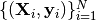, where each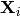is a multivariate sequence with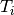samplesand each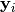target is a univariate sequence withsamples. The targetscan either be sequences of categorical class labels (for classification problems), or sequences of continuous data (for regression problems). The number of samplesvaries between the sequence pairs in the data set. Time series’ with a regular sampling period may be treated equivalently to sequences. Irregularly sampled time series are formulated with an additional sequence variable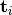that increases monotonically and indicates the timing of samples in the data set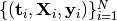.

Important sub-classes of the general sequence learning problem are sequence classification and sequence prediction. In sequence classification problems (eg song genre classification), the target for each sequence is a fixed class label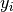and the data takes the form. Sequence prediction involves predicting a future value of the target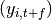or future values, given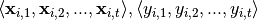, and sometimes also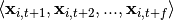.

A final important generalization is the case where contextual data associated with each sequence, but not varying within the sequence, exists to support the machine learning algorithm performance. Perhaps the algorithm for reading electrocardiograms will be given access to laboratory data, the patient’s age, or known medical diagnoses to assist with classifying the sequential data recovered from the leads.

seglearn provides a flexible, user-friendly framework for learning time series and sequences in all of the above contexts. Transforms for sequence padding, truncation, and sliding window segmentation are implemented to fix sample number across all sequences in the data set. This permits utilization of many classical and modern machine learning algorithms that require fixed length inputs. Sliding window segmentation transforms the sequence data into a piecewise representation (segments), which is particularly effective for learning periodized sequences. An interpolation transform is implemented for resampling time series’. The sequence or time series data can be learned directly with various neural network architectures, or via a feature representation which greatly enhances performance of classical algorithms.

## Why this Package¶

The algorithms to perform sliding window segmentation, padding & truncation, etc are straightforward. Without this package, pre-processing time series or sequence data to fixed length segments could be performed outside of the scikit learn framework, and the machine learning algorithms applied to the segments directly or a feature representation of them with scikit-learn. However, estimator performance is highly dependent on the hyper-parameters of the pre-processing algorithms (eg segmentation window length and overlap). seglearn lets you optimizing everything together.

The reason a new package is required to do this, instead of just a new transformer, is that time series segmentation and other transforms change the number of samples (instances) in the dataset. The final estimator sees one instance for each segment - which involves changing the number of samples and the target mid pipeline.

Changing the number of samples and the target vector mid-pipeline is not supported in scikit-learn - hence why this package is needed.

## What this Package Includes¶

The main contributions of this package are:

1. SegmentX - transformer class for performing the time series / sequence sliding window segmentation when the target is contextual

2. SegmentXY - transformer class for performing the time series / sequence sliding window segmentation when the target is a time series or sequence.

3. SegmentXYForecast - transformer class for performing the time series / sequence sliding window segmentation when the target is future values of a time series or sequence.

4. PadTrunc - transformer class for fixing time series / sequence length using a combination of padding and truncation

5. Interp - transformer class for resampling time series data

6. InterpLongToWide - transformer class for interpolating long format time series to wide format used by seglearn

7. FeatureRep - transformer class for computing a feature representation from segment data

8. FeatureRepMix - transformer class for computing feature representations where a different FeatureRep can be applied to each time series variable

9. Pype - sklearn compatible pipeline class that can handle transforms that change X, y, and number of samples

10. TS_Data - an indexable / iterable class for storing time series & contextual data

11. split - a module for splitting time series or sequences along the temporal axis

## What this Package Doesn’t Include¶

For now, this package does not include tools to help label time series data - which is a separate challenge.

## Valid Sequence Data Representations¶

Time series data can be represented as a list or array of arrays as follows:

>>> from numpy import array
>>> from numpy.random import rand

>>> # multivariate time series data: (N = 3, variables = 5)
>>> X = [rand(100,5), rand(200,5), rand(50,5)]
>>> # or equivalently as a numpy array
>>> X = array([rand(100,5), rand(200,5), rand(50,5)])


The target, as a contextual variable (again N = 3) is represented as an array or list:

>>> y = [2,1,3]
>>> # or
>>> y = array([2,1,3])


The target, as a continous variable (again N = 3), will have the same shape as the time series data:

>>> y = [rand(100), rand(200), rand(50)]


The TS_Data class is provided as an indexable / iterable that can store time series & contextual data:

>>> from seglearn.base import TS_Data
>>> Xt = array([rand(100,5), rand(200,5), rand(50,5)])
>>> # create 2 context variables
>>> Xc = rand(3,2)
>>> X = TS_Data(Xt, Xc)


TS_Data can be initialized from a pandas dataframe using column ‘ts_data’ for the time series:

>>> import pandas as pd
>>> df = pd.DataFrame(Xc)
>>> df['ts_data'] = Xt
>>> X = TS_Data.from_df(df)


There is a caveat for datasets that are a single time series. For compatibility with the seglearn segmenter classes, they need to be represented as a list:

>>> X = [rand(1000,10)]
>>> y = [rand(1000)]


If you want to split a single time series for train / test or cross validation - make sure to use one of the temporal splitting tools in split. If you have many time series in the dataset, you can use the sklearn splitters to split the data by series. This is demonstrated in the examples.

Irregularly sampled long format time series data (with timestamps) can be interpolated and transformed to wide format used by seglearn using the InterpLongToWide transformer:

>>> Xlong = pd.DataFrame({'time': np.arange(20), 'sensor': np.random.choice([1,2,3], 20), 'value': np.random.rand(20)})
>>> interp = InterpLongToWide(sample_period=1.0, kind='linear', assume_sorted=False)
>>> Xwide, _ , _ = interp.transform([Xlong.values])
`

Interpolation like this can be incorporated into a seglearn pipeline

## Using Seglearn¶

The package is relatively straightforward to use.

First see the Examples

If more details are needed, have a look at the API Documentation.